### Python实现12种概率分布（附代码）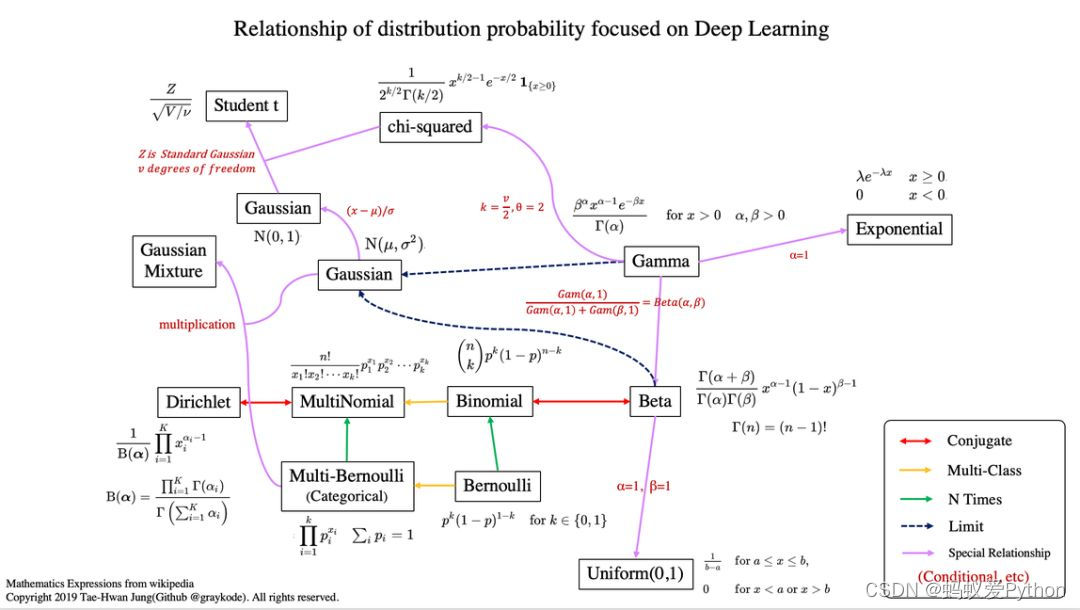``````Python学习交流Q群：906715085###

``````
• 1
• 2
• 3
• 4
• 5
• 6
• 7
• 8
• 9

## 1. 均匀分布（连续型）## 2. 伯努利分布（离散型）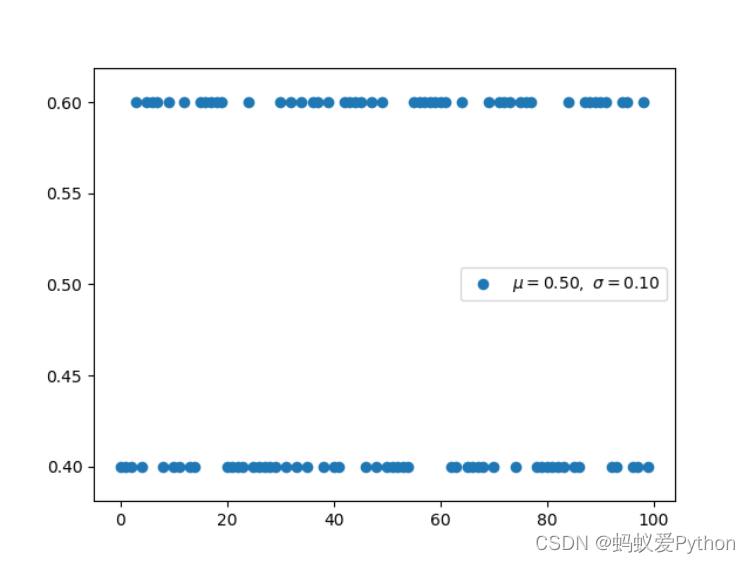## 3. 二项分布（离散型）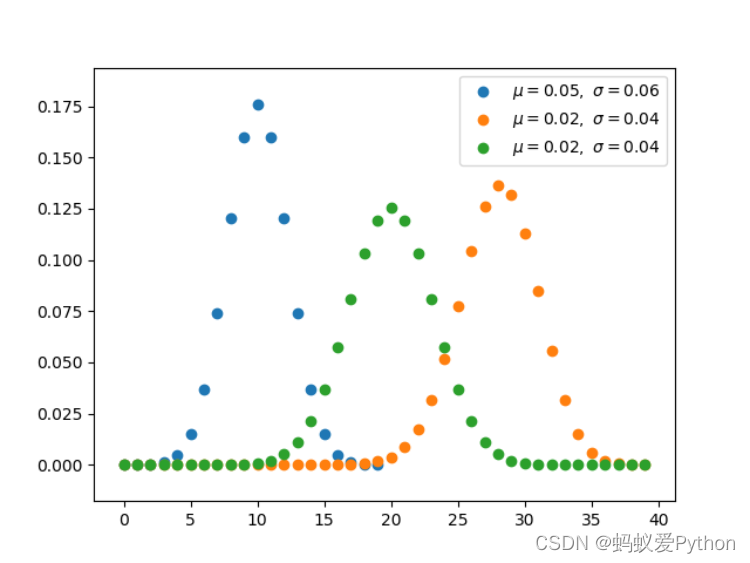## 4.Multi-Bernoulli 分布（离散型）

Multi-Bernoulli 分布又称为范畴分布（Categorical distribution），它的类别超过 2，交叉熵的形式与该分布的负对数形式是一致的。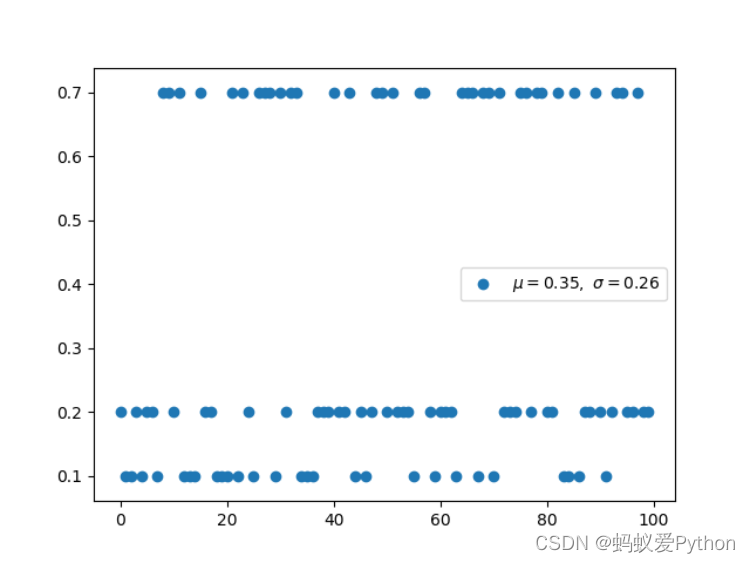## 5. 多项式分布（离散型）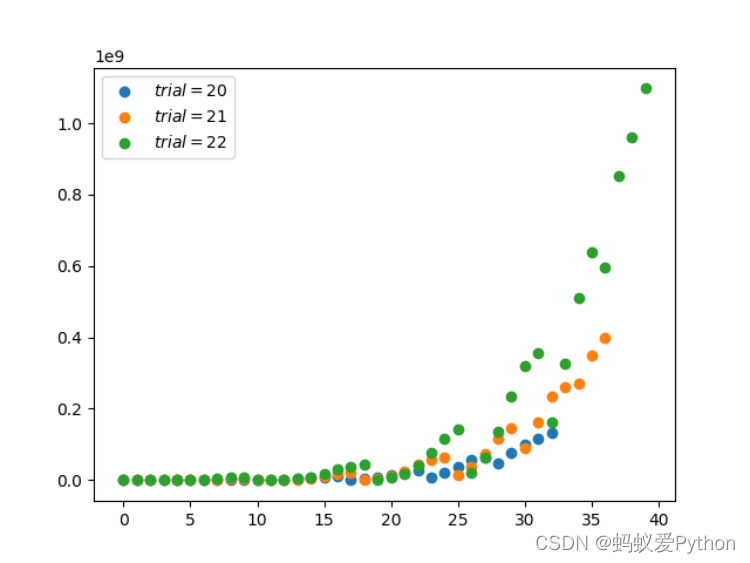## 6.Beta 分布（连续型）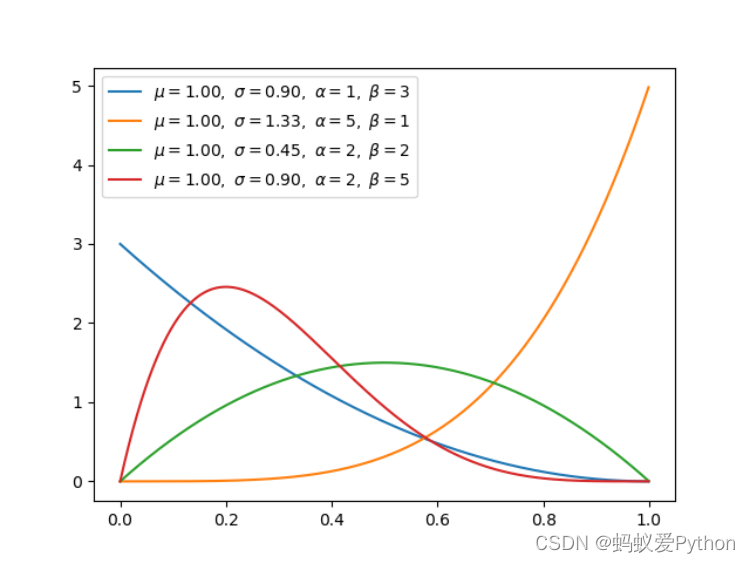## 7. 狄利克雷分布（连续型）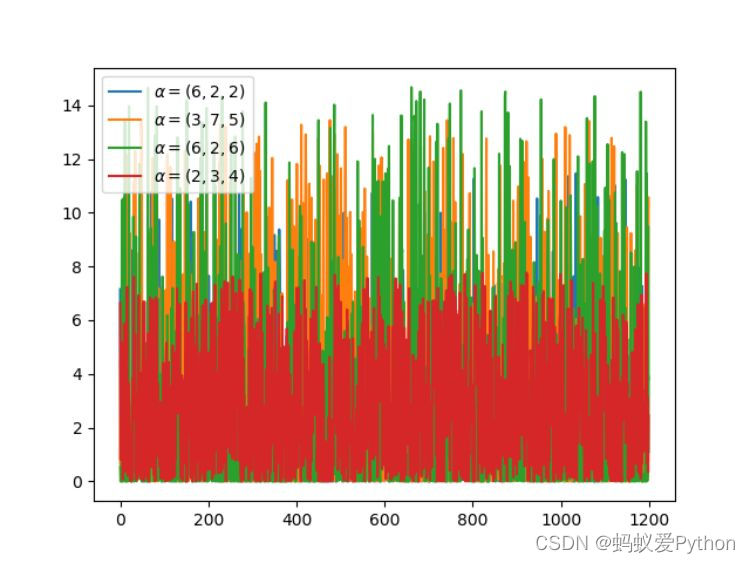## 8.Gamma 分布（连续型）

Gamma 分布是统计学中的常见连续型分布，指数分布、卡方分布和 Erlang 分布都是它的特例。如果 Gamma(a,1) / Gamma(a,1)

• Gamma(b,1)，那么 Gamma 分布就等价于 Beta(a, b) 分布。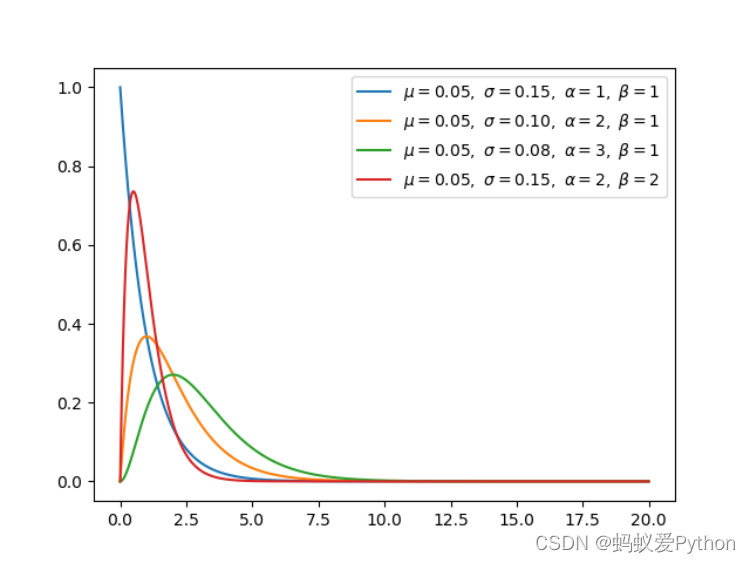## 9. 指数分布（连续型）

alpha 等于 1 时，指数分布就是 Gamma 分布的特例。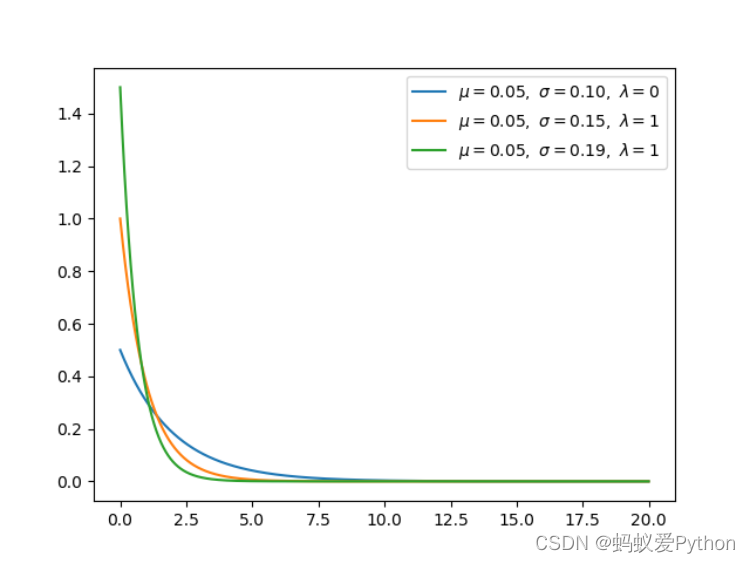## 10. 高斯分布（连续型）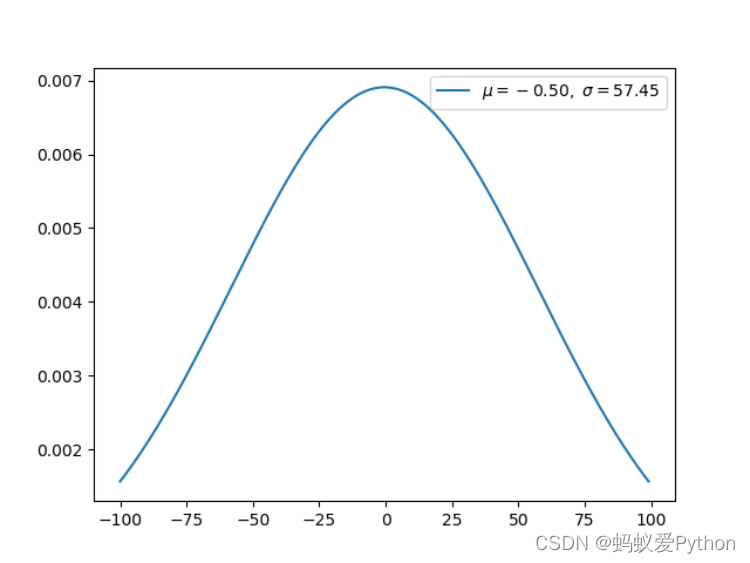## 11. 卡方分布（连续型）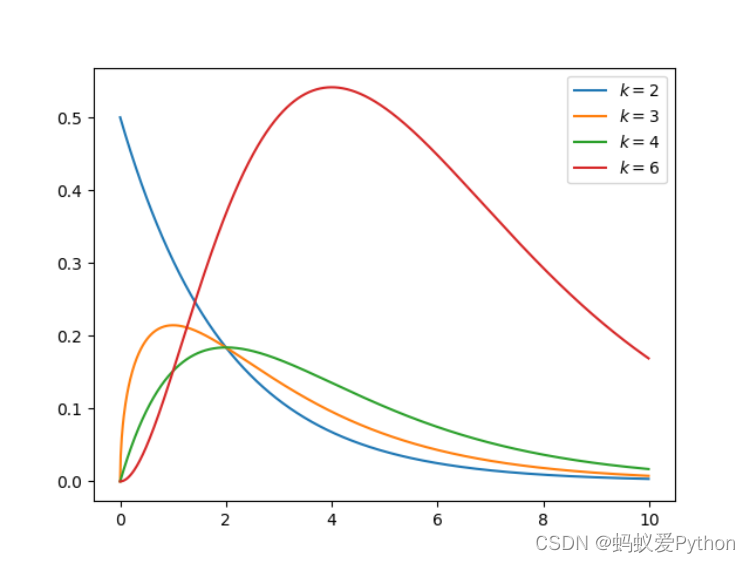## 12. 学生 t-分布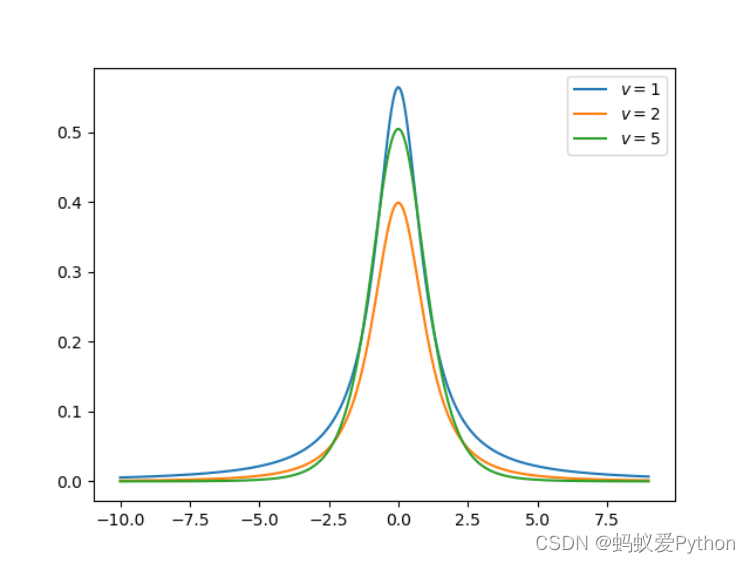``````import numpy as np
from matplotlib import pyplot as plt

def exponential(x, lamb):
y = lamb * np.exp(-lamb * x)
return x, y, np.mean(y), np.std(y)

for lamb in [0.5, 1, 1.5]:

x = np.arange(0, 20, 0.01, dtype=np.float)
x, y, u, s = exponential(x, lamb=lamb)
plt.plot(x, y, label=r'\$mu=%.2f, sigma=%.2f,'
r' lambda=%d\$' % (u, s, lamb))
plt.legend()
plt.savefig('graph/exponential.png')
plt.show()
``````
• 1
• 2
• 3
• 4
• 5
• 6
• 7
• 8
• 9
• 10
• 11
• 12
• 13
• 14
• 15
• 16

## 最后